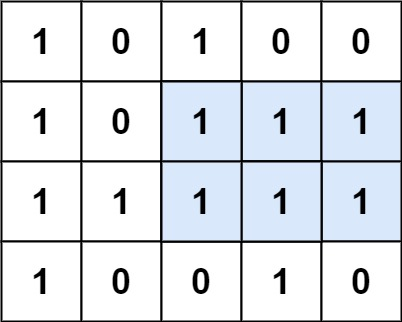### LeetCode• ㊗️
• 大家
• offer
• 多多！

## Problem

Given a rows x cols binary matrix filled with 0’s and 1’s, find the largest rectangle containing only 1’s and return its area.

Example 1:Input: matrix = [["1","0","1","0","0"],["1","0","1","1","1"],["1","1","1","1","1"],["1","0","0","1","0"]]
Output: 6
Explanation: The maximal rectangle is shown in the above picture.


Example 2:

Input: matrix = [["0"]]
Output: 0


Example 3:

Input: matrix = [["1"]]
Output: 1


## Code

class Solution {
public int maximalRectangle(char[][] matrix) {
if(matrix == null || matrix.length == 0) return 0;

int m = matrix.length;
int n = matrix.length;

int[] heights = new int[n];
int res = 0;

for(int i = 0; i < m; i++){

Stack<Integer> stack = new Stack<>();

for(int j = 0; j <= n; j++){

if(j < n){
if(matrix[i][j] == '1'){
heights[j] += 1;
} else {
heights[j] = 0;
}
}

int height = j == n ? 0 : heights[j];

while(!stack.isEmpty() && height <= heights[stack.peek()]){
int tempHeight = heights[stack.pop()];
int start = stack.isEmpty() ? -1 : stack.peek();
int area = (j - start - 1) * tempHeight;
res = Math.max(res, area);
}

stack.push(j);
}
}

return res;
}
}